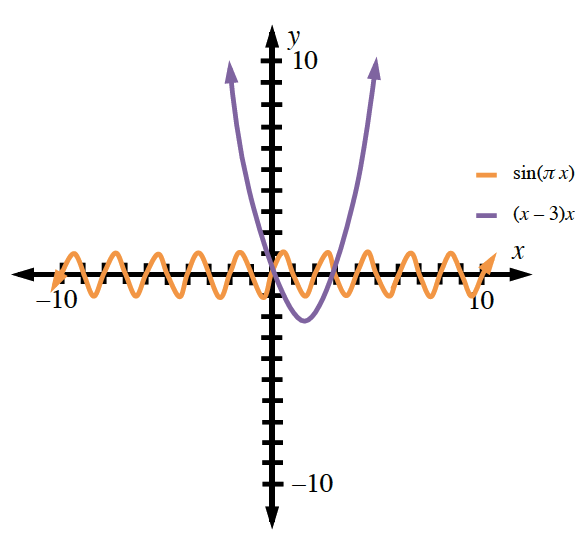### Home > APCALC > Chapter 5 > Lesson 5.1.3 > Problem5-28

5-28.

Calculate the area between the curves $y=\sin\left(\pi x\right)$ and $y = x^{2} – 3x$.

Use your calculator to find the points of intersection between the two graphs.
Then determine which graph is on top (has higher $y$-values) and which is below.$\text{integrate:}\int_{\text{intersection }A}^{\text{intersection }B}([\text{top function}]-[\text{bottom function}])dx$

$5.137$ units2